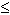DOC HOME SITE MAP MAN PAGES GNU INFO SEARCH PRINT BOOK

# frexp(S)

frexp, frexpf, frexpl, ilogb, ilogbf, ilogbl, ldexp, ldexpf, ldexpl, logb, logbf, logbl, modf, modff, modfl, nextafter, nextafterf, nextafterl, scalb, scalbf, scalbl -- manipulate parts of floating-point numbers

## Synopsis

```   #include <math.h>

double frexp(double value, int *eptr);

float frexpf(float value, int *eptr);

long double frexpl(long double value, int *eptr);

int ilogb(double value);

int ilogbf(float value);

int ilogbl(long double value);

double ldexp(double value, int exp);

float ldexpf(float value, int exp);

long double ldexpl(long double value, int exp);

double logb(double value);

float logbf(float value);

long double logbl(long double value);

double nextafter(double value1, double value2);

float nextafterf(float value1, float value2);

long double nextafterl(long double value1, long double value2);

double scalb(double value, double exp);

float scalbf(float value, float exp);

long double scalbl(long double value, long double exp);

double modf(double value, double *iptr);

float modff(float value, float *iptr);

long double modfl(long double value, long double *iptr);
```

## Description

Every non-zero number can be written uniquely as x*2^n, where the ``mantissa'' (fraction) x is in the range 0.5| x | < 1.0, and the ``exponent'' n is an integer. frexp returns the mantissa of a double value and stores the exponent indirectly in the location pointed to by eptr. If value is zero, both results returned by frexp are zero. frexpf returns the mantissa of a float value. frexpl returns the mantissa of a long double value.

ilogb, ilogbf and ilogbl return the (unbiased) exponent part of value. It is equivalent to (int)logb[f|l](value).

ldexp, ldexpf, ldexpl, scalb, scalbf and scalbl return the quantity value*2^exp.

logb returns the unbiased exponent of its floating-point argument as a double-precision floating-point value.

logbf returns the unbiased exponent of its floating-point argument as an float-precision floating-point value.

logbl returns the unbiased exponent of its floating-point argument as an extended double-precision floating-point value.

modf, modff and modfl return the signed fractional part of value and store the integral part indirectly in the location pointed to by iptr.

nextafter, nextafterf and nextafterl return the next representable floating-point value following value1 in the direction of value2. Thus, if value2 is less than value1, nextafter, nextafterf and nextafterl return the largest representable floating-point number less than value1.

### Errors

If value is 0 or NaN, ilogb, ilogbf and ilogbl return INT_MIN. If value is ± infinity, ilogb, ilogbf and ilogbl return INT_MAX.

If the correct value of ldexp, ldexpf, ldexpl, scalb, scalbf or scalbl would cause overflow, these functions return a value that will compare equal to ±HUGE_VAL (according to the sign of value) and set errno to ERANGE. If the correct value of these functions would cause underflow, zero is returned and errno is set to ERANGE.

logb or logbl of zero returns -HUGE_VAL; on systems that support IEEE floating-point, the divide by zero exception is raised. On systems that support IEEE floating-point, scalb, scalbf, scalbf, logb, logbf or logbl of ± infinity returns + infinity. For both of these error cases, errno is set to EDOM.

On systems that support IEEE floating-point, if input value1 to nextafter, nextafterf or nextafterl is ± infinity, that input is returned and errno is set to EDOM. The overflow and inexact exceptions are signaled when input value1 is finite, but

```   nextafter(value1, value2)
```

is not. The underflow and inexact exceptions are signaled when the returned value is denormalized. In both cases errno is set to ERANGE. (These last two conditions apply to nextafterl, as well.)

On systems that support IEEE NaN, if the input to any of these functions is a quiet NaN, that NaN is returned. If the input is a signaling NaN, a quiet NaN is returned and the invalid operation exception is raised. In either case, errno is set to EDOM.

When the program is compiled with the cc option -Xt [see cc(C)], the returned value of ldexp, ldexpl, scalb, scalbf and scalbl will compare equal to HUGE instead of HUGE_VAL.

cc(C), intro(S)